>

## NCERT Solutions for Class 10 Maths Chapter 15 Probability Exercise 15.1

Here we have provided Chapter 15 Probability Exercise 15.1 NCERT Solutions for Class 10 Maths that will be helpful in understanding the basics of the chapter as well as completing your homework with accuracy. NCERT Solutions given here will be very useful in improving your marks in the examinations. Class 10 Maths Solutions are also beneficial in boosting your efficiency of problem solving. By taking help from these solutions, you can take frame your own answers and boost your morale.

There is only one exercise in this chapter, Exercise 15.1 which have total 25 questions based on finding probability of occurring of an event.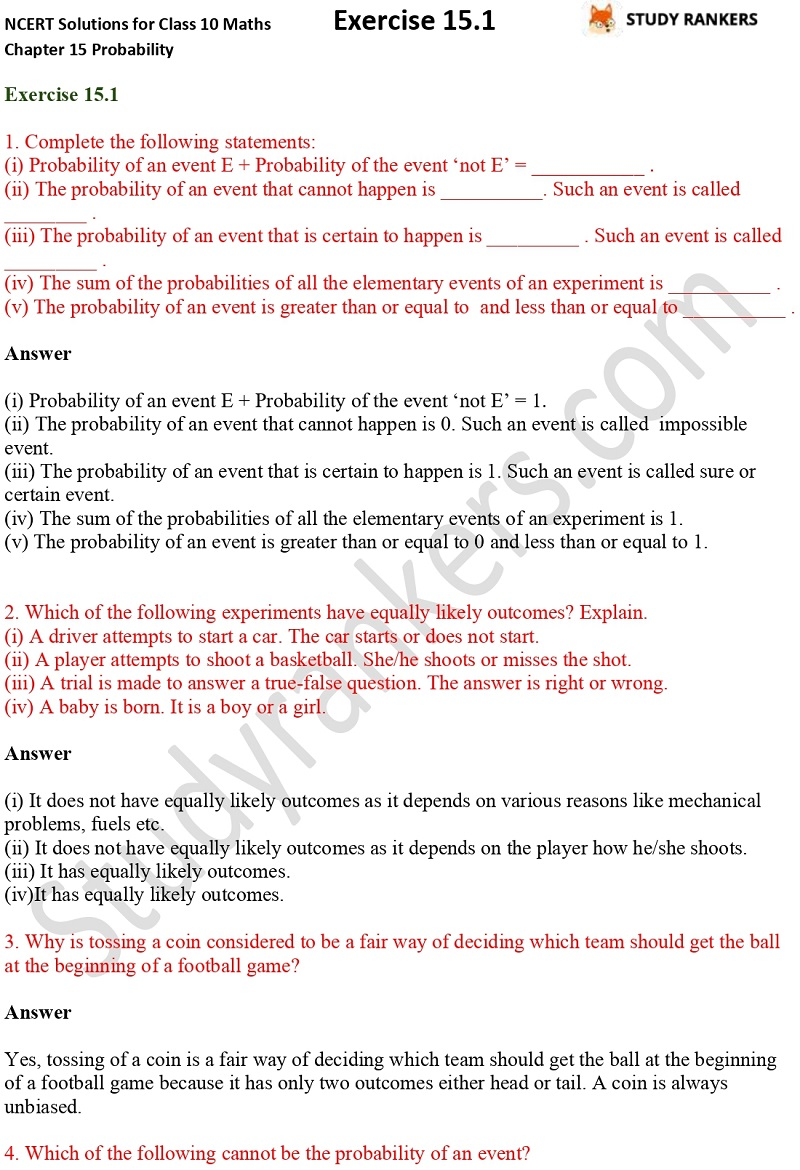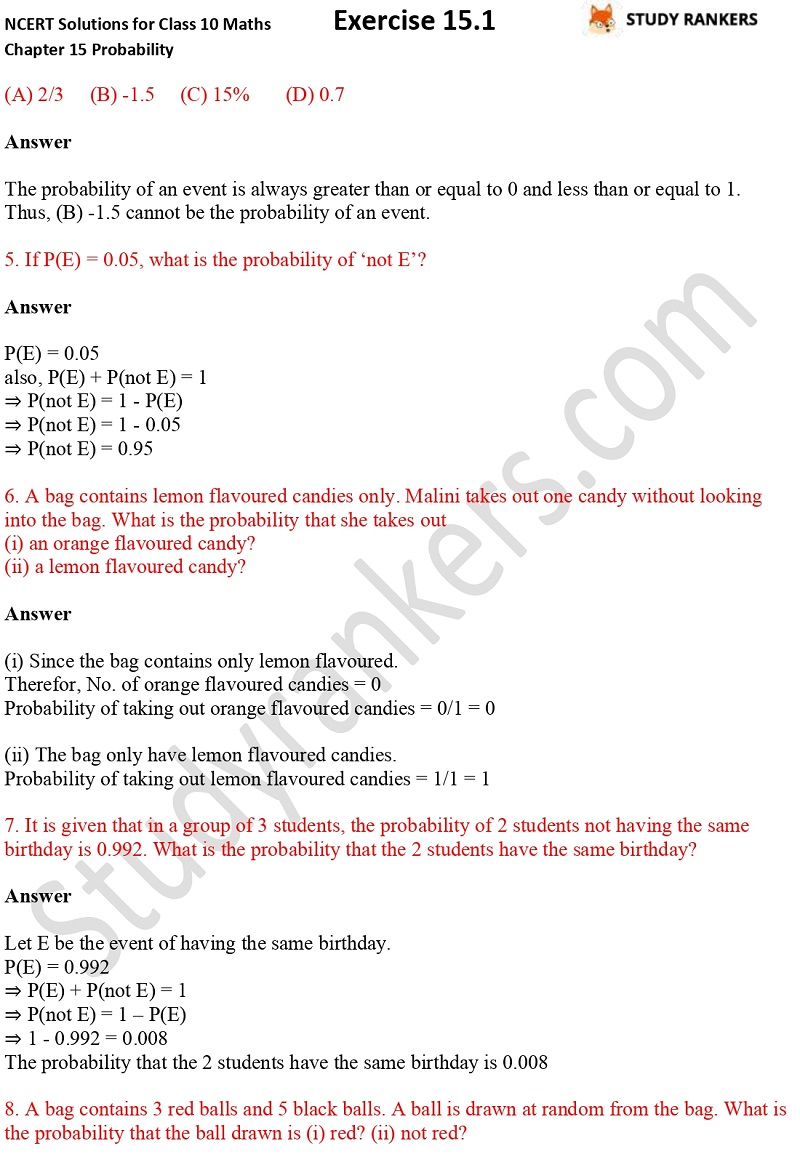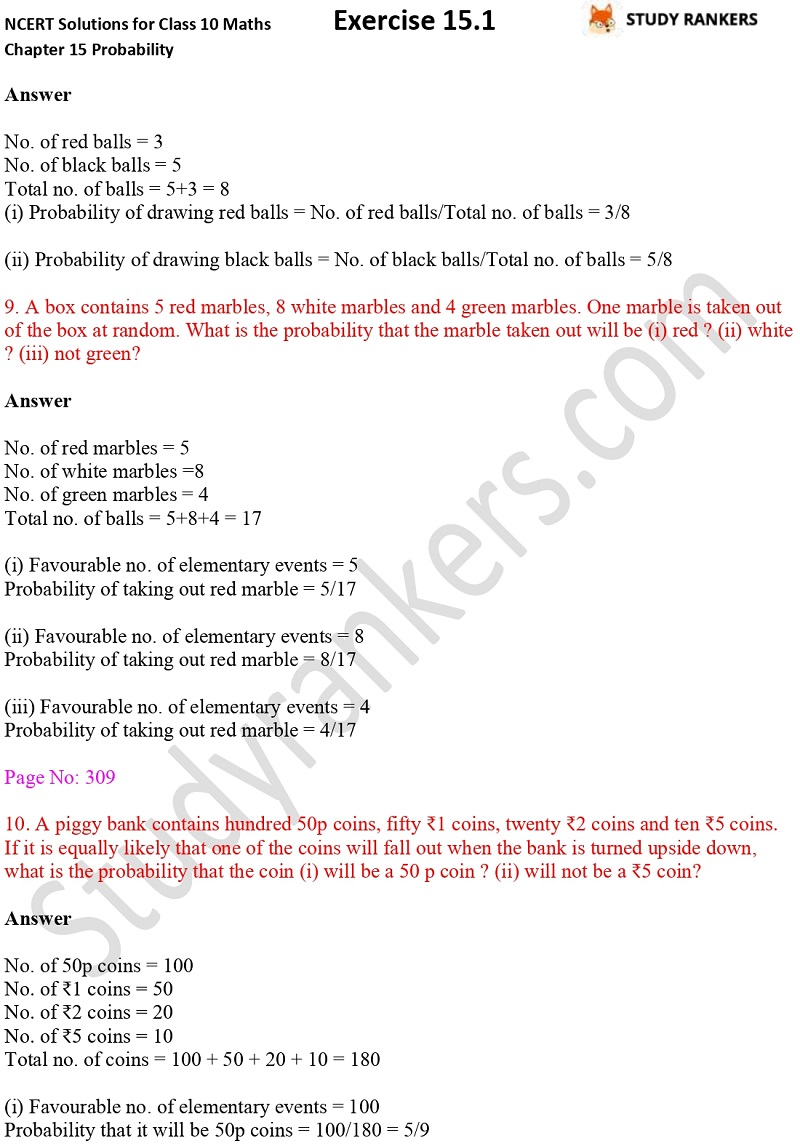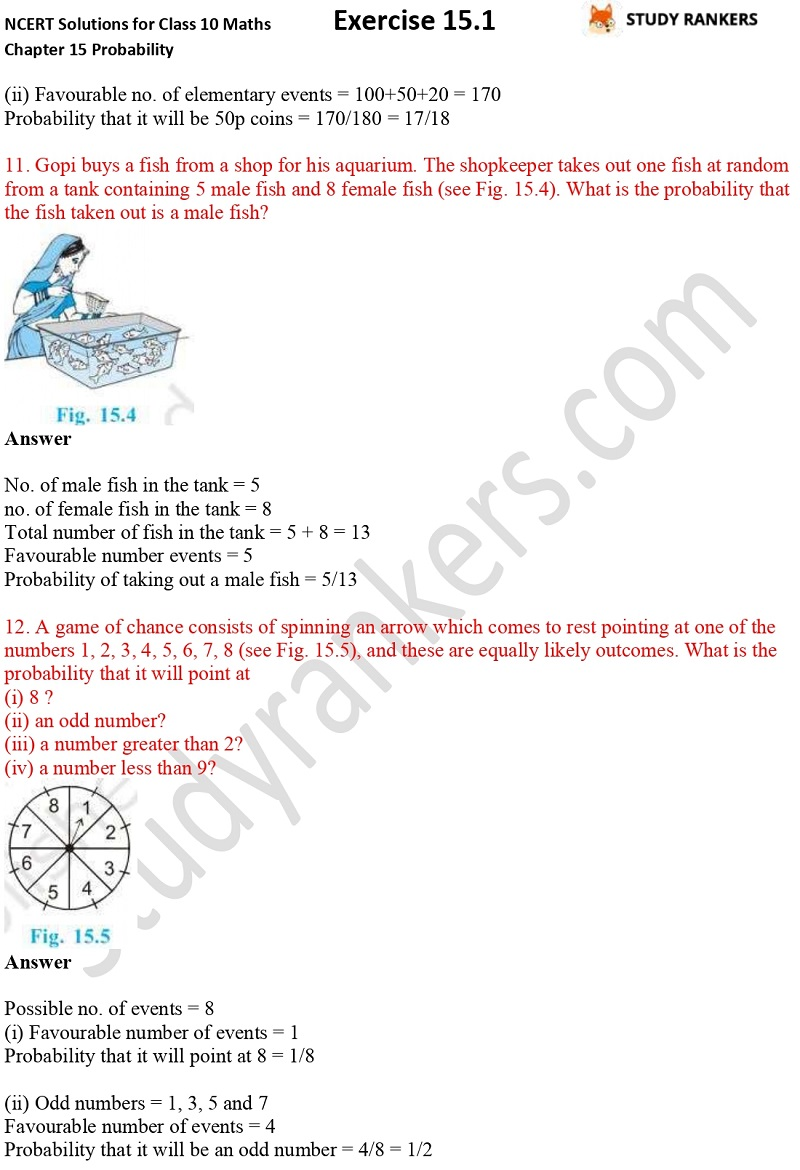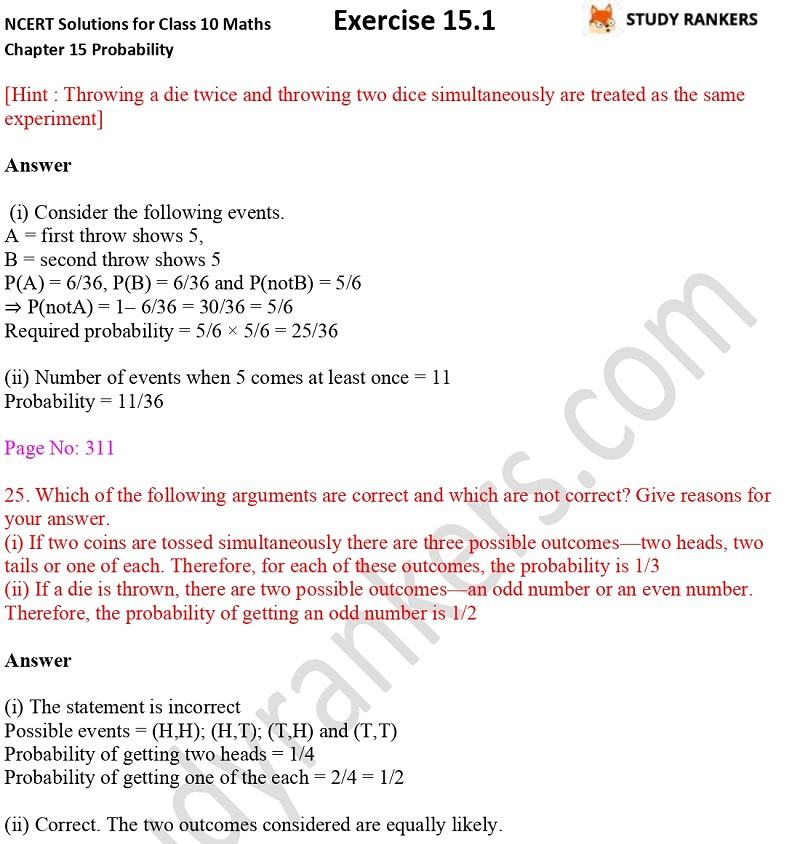X